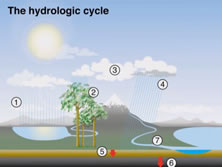You haven't completed the exercise.

# Review unit (Exercise 3.5)Figure 1

1. What kind of visual aid does the presenter use?

2. Look at Figure 1. If you were to label the diagram with words instead of numbers, which term would replace number 1?

3. Which number would condensation replace?

4. Which term would replace number 5?

5. Which number would run-off replace?